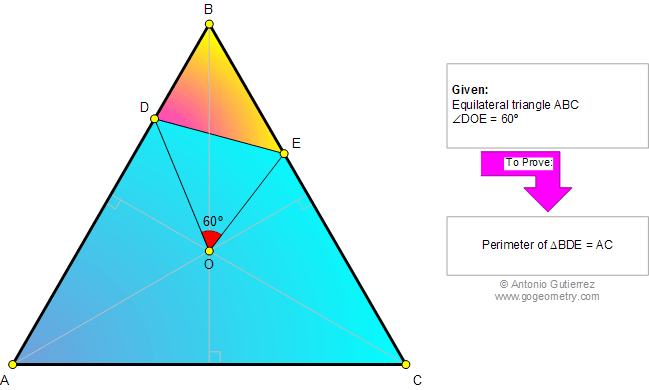# Online Geometry Problem 754: Equilateral Triangle, Center, Angle, 60 Degrees, Perimeter. Level: High School, College, Mathematics Education

 The figure shows an equilateral triangle ABC with center O. D and E are on AB and BC, respectively, so that angle DOE = 60 degrees. Prove that the perimeter of triangle BDE is equal to the measure of AC. This entry contributed by Ajit Athle.Home | Search | Geometry | Problems | All Problems | Open Problems | Visual Index | 751-760 | Triangles | Equilateral Triangle | 60 degrees | Perimeter | Triangle Center | Email | Solution / comment | By Antonio Gutierrez